# 2.7: Solving ODEs with Mathematica- How to find numerical and analytical solutions to ODEs with Mathematica

•• Contributed by Peter Woolf et al.
• Assistant Professor (Chemical Engineering) at University of Michigan

Authors: Matthew Baumgartner, Olyvia Dean, Viral Patel, Joel Schweitzer, and Eric Van Beek

Stewards: Brian Hickner, Lennard Gan, Addison Heather, Monique Hutcherson

Date Released: September 6, 2006 /Date Revised: September 8, 2007

## 7.1 Introduction

Mathematica is an advanced mathematics solution program created by Wolfram Research, Inc. One of the most powerful software packages of its kind, Mathematica is able to easily and conveniently solve complicated mathematical formulae, including differential equations. This article focuses on the modeling of first and higher order Ordinary Differential Equations (ODE) in the following forms:

$\frac{d y}{d x}=f(x, y) \tag{Basic ODE}$

$\frac{d y^{z}}{d^{z} x}=f(x, y) \tag{Higher Order ODE}$

Like all software, Mathematica uses a specific language in order to perform calculations. The names of all functions must be capitalized- this applies to both mathematical functions (such as Sin and Cos) and built-in functions (such as Plot and DSolve). For this reason it is common for users to write their own functions in minimized letters. This decreases the chance of overriding or redefining a Mathematica function. Square brackets always follow function calls, and the function's parameters are always enclosed in curved brackets. For example, if a user wished to plot sin(x) from x = 0 to x = 1, they would type: Plot[Sin[x],{x,0,1}]. The user must type "Shift"+"Enter" to input the function. Typing only "Enter" will add a line to the formula. . For full PC keyboards, the “Enter” key on the far right is the equivalent of “Shift”+”Enter”. If the user does not want to see the output line, it can be suppressed by typing a semi-colon at the end of the expression. Mathematica also recognizes common mathematical constants such as pi (Pi), Euler's constant (E), and imaginary numbers (I). Note that these constants must also be capitalized when entered.

Mathematica features two functions for solving ODEs: DSolve and NDSolve. DSolve is used when the user wishes to find the general function or functions which solve the differential equation, and NDSolve is used when the user has an initial condition. The prompts for the functions are quite similar. Note: Two equal signs must be used to denote equality in an equation. Using one equal sign assigns a value to a variable.

Example:

f(x) = 5x2 + 7 This input creates a variable named f(x) that has a value of 5x2 + 7.

f(x) = = 5x2 + 7 This input creates a function f(x) which is defined by 5x2 + 7.

Mathematica will sometimes store user created functions or variables into its cache. This causes some (potentially) correct commands to output errors. It is recommended to quit the local kernel and reinitialize the commands after any changes to your code.Also, check that every command in the code is being executed. Clearing all the outputs in the cell may be helpful to figure out which commands had not been executed. This can be done by going to the “Cell” option found on the top and choosing the “Delete All Output” Example:

OUTPUT IS NOT CLEAREDCLEARING THE OUTPUTS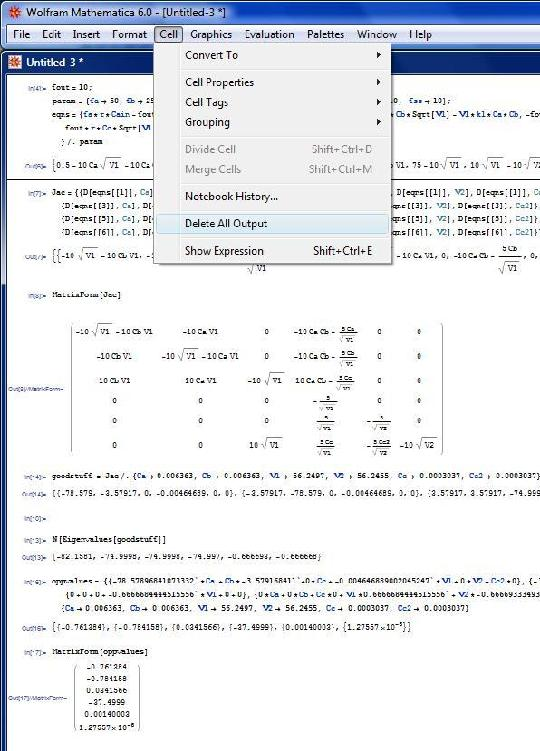CODE WITH CLEARED OUTPUTSAs seen in the examples, it is easier to troubleshoot and debug your program when it looks less confusing. Clearing the extra outputs helps you focus on just the code that you have written.

## 7.2 First Order ODEs

Notation: In the following examples, eqn represents the ODE, y represents the function being solved for, i represents the initial condition, and x and t are independent variables.

### 7.2.1 ODEs With Initial Conditions

NDSolve will not display a numerical value, but rather an “Interpolating Function” which can be displayed graphically. The easiest way to display this graph is to assign the solution to a variable (called Solution in the example) in the input line, and then use the “Plot” function to display it.

Input for One ODE: Solution = NDSolve[{eqn,y == i},y,{x,xmin,xmax}]

Input for Multiple ODEs: Solution = NDSolve[{eqn1,eqn2,...,y1 == i1,y2 == i2,...},{y1,y2,...},{x,xmin,xmax}]

Input for Partial Differential Equations: Solution = NDSolve[{eqn,y == i},y,{x,xmin,xmax},{t,tmin,tmax}]

After Mathematica solves the ODE, plot the solution by typing: Plot[Evaluate[y[x] /. Solution],{x,xmin,xmax}], where “/.” is a notation used by Mathematica meaning “following the rule of”. It basically recalls the function stored as the variable in the previous input line. Depending on the needs of the user, the functions “ParametricPlot” or “Plot3D” may also be useful. The notation for these functions is the same as “Plot”.

Here is a simple example of what you would type into Mathematica:

• Solution = NDSolve[{y'[x]==y[x]*Cos[x+y[x]],y==1},y,{x,0,30}]

Mathematica will output:

• Output= {{y->InterpolatingFunction[ { { 0.,30.} },<>] } }

To plot this function you would type:

• Plot[Evaluate[y[x]/.Solution],{x,0,30}]

Note: Remember to type "Shift"+"Enter" to input the function

### 7.2.2 ODEs Without Initial Conditions

Input for One ODE: DSolve[eqn,y,x]

Input for Multiple ODEs: DSolve[{eqn1,eqn2,…},{y1,y2,…},x]

Input for a Partial Differential Equation: DSolve[eqn,y,{x1,x2,…}]

Here is a simple example of what you would type into Mathematica:

• Solution = DSolve[y'[x] ==4*x-2*x*y[x],y[x],x]

Mathematica will output:

• Output=
ParseError: invalid Primary (click for details)
Callstack:
at (Bookshelves/Chemical_Engineering/Chemical_Process_Dynamics_and_Controls/02:_Modeling_Basics/2.07:_Solving_ODEs_with_Mathematica-_How_to_find_numerical_and_analytical_solutions_to_ODEs_with_Mathematica), /content/body/div/div/ul/li/span, line 1, column 6


## 7.3 Second Order and Higher ODEs

Input for Higher Order ODEs: Solution = NDSolve[{eqn,y == i1, y'  == i2,…},y,{ x,xmin,xmax}]

Plotting the solution: Plot[Evaluate[{y[x], y' [x],...} /. Solution],{x,xmin,xmax}]

Here is a simple example of what you would type into Mathematica:

• Solution = NDSolve[{y[x] +Sin[y[x]]+y[x]==0,y==1,y'==0,y,{x,0,30}]

Mathematica will output:

• Output= {{y->InterpolatingFunction[ { {0.,30.} },<>] } }

To plot this function you type:

• Plot[Evaluate[{y[x],y'[x],y[x]}/.Solution],{x,0,30}]

Mathematica should output a graph.

## 7.4 Algorithms Used by Mathematica

Mathematica uses two main algorithms in order to determine the solution to a differential equation. These algorithms are the Adams method and the Gear method.

The Adams and Gear methods are forms of linear multistep methods. An example of these would be the following: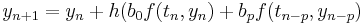In the example above, h denotes the step size and the coefficientsare determined by the method used. Multistep methods are expansions of more familiar single-step methods used to solve differentials (i.e. Euler, Runge-Kutta, Taylor). Each of these methods requires an initial point in order to calculate the following point. Similarily, multistep methods also require initial points in order to solve the ODE. The number of initial points required depends on which method is used to solve the ODE. Multistep methods typically produce less error than the single-step methods because of multiple initial points.

In order to determine what method to use one must first find the stiffness of the function.

Euler, Taylor and Runge-Kutta methods used points close to the solution value to evaluate derivative functions. The Adams-Bashforth method looks at the derivative at old solution values and uses interpolation ideas along with the current solution and derivative to estimate the new solution . In order to solve an ODE using this method, f(t,y) must be continuous and satisfy Lipschitz condition for the y-variable which states :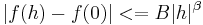for all | h | < ε where B and β are independent of h, β > 0 and α is an upper bound for all β for which a finite B exists

This is a basic form of the Adams-Bashforth method. Note that two initial points are required for this method.There is another Adams method that requires three initial points. The method is solved the same way, however the equation varies a little bit and is referred to as the Adams-Moulton Method.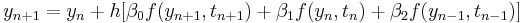The coefficients/constraints, β can be solved for using knowledge of ODE's and other math tools. It was stated earlier that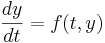. We can let f(t,y) = λy, therefore. We can also letif there is a constant step size and σ represents a polynomial. Through substitution we find :We can expand the quadratic using another math identity and ultimately solve for constraints β1 and β2. Another method for solving for coefficients β12 is mentioned below:

In order to find the coefficient βj one must first use polynomial interpolation to find the polynomial p of degree s − 1 such that:forFrom this the Lagrange formula for polynomial interpolation yieldsNow the polynomial p is a locally good approximation of the right-hand side of the differential equation y' = f(t,y) that is to be solved. Now we must consider the equation y' = p(t) instead. This equation can be solved exactly by simply taking the integral of p.The Adams–Bashforth method arises when the formula for p is substituted. The coefficients bj turn out to beThe Adams-Bashforth method is typically used for Linear and Non-liner ODE's with dense systems.

### 7.4.2 Gear Method

Takingfor i ≥ 1

The Gear method, also known as the backward differentiation formulae (BDF, a.k.a. Gear’s formulae) is another multi-step method but is generally used for multiple equations. In order to use the gear method your function must have a stiffness greater than 500, meaning that the function is stiff.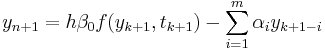As a particular cases, takingfor i ≥ 2 and optimizing the remaining coefficients to maximize the accuracy of the resulting scheme recovers the Implicit Euler method. Takingfor i ≥ 3 givesWe now focus on this case in particular. Applying this method to the scalar model problemand assuming constant h and a solution of the form, we find the following quadratic equation forthe two roots of which are given by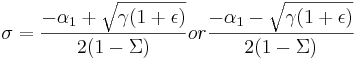Applying the identities, we may expand both roots in terms of powers of h. By our assumed form of the solution, it follows that.The leading-order term in the expansion in h of(a “spurious root”) is proportional to h. For small h,quickly decays to zero, and thusmay be neglected. The leading-order terms in the expansion in h of(the “physical root”) resemble the Taylor-series expansion of the exact solution over a single timestep.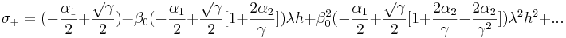Matching coefficients with the expansion of the exact solution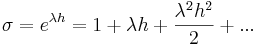as indicated by underbraces in the above expression, and applying the definition, we arrive at three equations forto achieve the highest order of accuracy possible with this form. It is easily verified that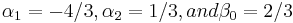satisfy these three equations. The leading-order error term of this method is proportional to. Thus, over a single timestep, the scheme is “locally third-order accurate”; more significantly, over a fixed time interval [0,T], the scheme is globally second-order accurate. The resulting method,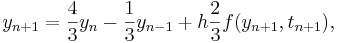is thus referred to as BDF2, and may be viewed as a implicit alternative to AM3 that, for the same number of steps into the past, p = max(m,n), has reduced order of accuracy but greatly improved domain of stability. Higher-order BDFs may be derived in an analogous fashion; BDF3, BDF4, BDF5, and BDF6 in particular are found to have excellent stability properties as compared with their AM counterparts with the same number of steps.

## 7.5 Worked out Example 1

You have been assigned a non-isothermal reactor with the following material and enthalpy balances.Species a Material balance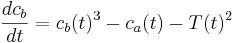Species b Material balanceEnthalpy balance

When is the concentration of species a and species b equal? The initial conditions for the reactor are as follows:

T0 = 1

ca0 = 2.0

cb0 = 1.8

tf = 0.2

s = NDSolve[{x'[t] == x[t]^2 - y[t] - z[t]^2, y'[t] == y[t]^3 - x[t] - z[t]^2, z'[t] == x[t] - y[t] - z[t]^2, x == 2, y == 1.8, z == 1}, {x, y, z}, {t, 0.2}]

Plot[Evaluate[{x[t],y[t]} /. s], {t, 0, 0.2}]## 7.6 Worked out Example 2

You have been asked to study a semi-batch reactor for the reaction. The rate law is, where k = 2.7. Other parameters are: initial volume = 5, volumetric flow rate = 0.05, initial concentration of A = 2500. Use Mathematica to create a Conversion versus Time plot for 100 time units.

1. Mole Balance:2. Rate Law:3. Stoichiometry:4. Combine:If the semi-batch reactor runs for 100 time units, the conversion is about 0.8. This can be seen in the plot below or by running the two commands that follow into Mathematica.

s = NDSolve[{y'[t] == 2.7 (1 - y[t]) (5 + .05 t)/(2500 y[t]), y == 0.0001}, y, {t, 0, 100}];

Plot[Evaluate[y[t] /. s], {t, 0, 100}, PlotRange -> All]This is the Mathematica notebook file for the example: Media:MathematicaEx2.nb

Note: The parameters used in this problem are fabricated and are intended to illustrate the use of Mathematica in solving ODEs.

## 7.7 Formatting Plots in Mathematica

Here are some useful tips to formatting plots in Mathematica:

Label Plot: e.g. Plot[Sin[x], {x,0,2Pi}, PlotLabel->"Volume of Tank vs. Time"]

Label Axes: e.g. Plot[Sin[x], {x,0,2Pi}, AxesLabel->{"time(min)","volume(L)"}]

Color Plot: e.g. Plot[Sin[x], {x,0,2Pi}, PlotStyle->Red]. You can also make the plot thick, dashed, etc. e.g. Plot[Sin[x], {x,0,2Pi}, PlotStyle->{Red,Thick,Dashed}]

Insert Legend: e.g. Needs["PlotLegends`"] <--MUST insert this BEFORE your plot command. Plot[{Sin[x],Cos[x]}, {x,0,2Pi}, PlotLegend->{"sine", "cosine"}]

## 7.8 Sage's Corner

Solving ODEs with Mathematica Video

slides for this talk

## 7.9 Additional Tips and Tricks for Troubleshooting in Mathematica

Mathematica is a powerful computing tool, however the syntax can be a bit difficult to understand. Here are some notes for troubleshooting in Mathematica.

1. Check to make sure that your variable names and signs are consistent.

• Ex) Make sure you use xI everywhere instead of xI and x1 or xl.
• Ex) Functions, including the ones you create, are usually followed by brackets such as Sin[x] or y[x]. However, brackets are not necessary when you are solving for a function in a set of differential equations such as NDSolve[eqns, {y}, {x, 0, 50}];
• Ex) Check to see if your parentheses are aligned such that you are actually entering the function you think you're entering. Recall order of operations and the distributive property. x*(a+b) is NOT equal (x*a) + b. This seems simple, but often gets overlooked when dealing with several lines of code.

2. You may find it easier to define all of your variables together at the beginning of your code. This makes it easy to go back and change the values assigned to each variable if trying to determine the impact one variable has on a system of equations. For instance, say you are trying to determine the effects the flow rates into two different tanks (F1, F2) will have on the tank volumes over ten time steps. The differential equations governing the situation are:and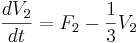, where F1 = 2, F2 = 12, V1(0) = 0, V2(0) = 0.

If you write the ODEs in Mathematica by directly substituting in F1 = 2 and F2 = 12, you will have to change the ODEs each time you change the values of F1 and F2. Below is an example of what the Mathematica equations would look like.

s = NDSolve[{V1’[t] == 8 – V1[t], V2’[t] == 12 – (1/3)*V2[t], V1 == 0, V2 == 0},{V1,V2},{t,0,10}]

Another option is to define F1 and F2 before your equations, and then call them when solving for your ODEs. This allows you to easily substitute in new values for your variables without changing the differential equations, and reduces the odds of making simple computational errors in your ODE in the process. The Mathematica code would look as shown below.

variables = {F1 -> 2, F2 -> 12};

s = NDSolve[{V1’[t] == 4*F1 – V1[t] , V2’[t] == F2 – (1/3)*V2[t], V1 == 0, V2 == 0} /. variables,{V1,V2},{t,0,10}]

3. Be Aware of the Kernel

The Mathematica Kernel stores all of the information about variable definitions. When you define a variable , the definition is stored there. The kernel is automatically started when a new Mathematica session is started. You may also start it manually. To start the kernel manually go to Evaluation -> Start Kernal -> Local. Once the kernel is started and you wish to go back and change a variable definition you must "Quit the Kernal" before you see the change occur. In order to "Quit the Kernal" you must go to Evaluation -> Quit Kernal -> Local. Terminating the Mathematica Kernal, erases all of the definitions previously entered. For this reason, after you "Quit the Kernal" and enter in new definitions for your variables you must re-enter all of your code. The images below show how to "Start Kernal" and how to "Quit the Kernel."Example:

An example of when you would want to "Quit the Kernal" when using Mathematica for Controls is when you are finding a steady state point using a PID controller. We start by defining the variables for vset, taui, taud, and Kc. Mathematica solves this equation using the defined variables and shows that (x->3.9, V->10) is the steady state point. This is shown in the figure 1 below.Figure 1 when you defined Kc even though you have not defined it here.Figure 3 shows the output once you have "Quit the Kernel" and re-entered the Mathematica code.Now you see that the definition for Kc has been deleted because the steady state points are in terms of Kc. To find the impact of Kc you can use this solution.

4. Define functions or formulas that are used often

If you will use a function often and it is not already defined in Mathematica by default, define your own. This can be especially helpful if you intend repeat the same function multiple times while only changing variables. A scenario where defining your own function could save you time and error is when comparing P-Fisher's values while keeping the margins constant. Only the variables will change but the function will remain the same. The new function will only last as long as each session in Mathematica.

To define a function, begin by naming the function followed by an open bracket. List all the variables with an underscore after each name and separate each variable with a comma. Close the bracket when all the variables have been listed. To let Mathematica know that this is a newly defined function, after closing the bracket place a semi-colon and equal sign. Now define what the formula is using the variable names. When you have finished, click shift-enter simultaneously. To test the new formula, start with naming the function and open bracket, then list all the variables numerical values separated by comma's in the same order that was listed when defining the function. Close the bracket after all the variables have been listed and click shift-enter simultaneously. The output will be the next line in Mathematica with the answer.

Example: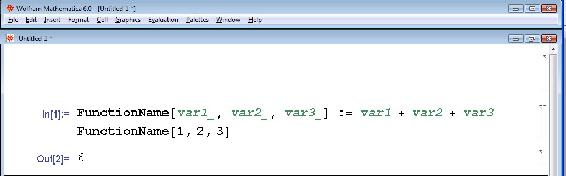It is also possible to use this tool to put equations in terms of unknown variables. To do this begin by defining the function the same way as before. When plugging in the numerical values of the variables, leave the unknown variables as their variable name. The Mathematica output will provide the answer in terms of the unknown variables.

Example:5. "Make it pretty"

Oftentimes when people program in any language, they tend to crowd lines, almost as if they were trying to save paper. Well, since this does not apply when you are typing on a screen, don't worry about it.

• Ex) You could write:

vars = {V -> 1000, Cao -> 0.2, UA -> 20000, F -> 2000, DE1 -> 30000, k1 -> 1.0 10^13, DH1 -> 50000, rcp -> 60, R -> 1.987, To -> 500, Tf -> 500, Tjin -> 500, Fj -> 1000, Vj -> 100, Caf -> 0.2, DH2 -> -192000, DE2 -> 30000, k2 -> 1.0 10^13};

eqns = {Ca'[t] == (Caf - Ca[t]))/V - Ca[t]*k1*Exp[-DE1/(R*T[t])], Cb'[t] == (0 - Cb[t])/V +k1*Ca[t]*Exp[-DE1/(R*T[t])] - k2*Cb[t]*Exp[-DE2/(R*T[t])], T'[t] == (Tf - T[t])/V + (-DH1/(rcp))*k1*Ca[t]*Exp[-DE1/(R*T[t])] + (-DH2/rcp )*k2*Cb[t]*Exp[-DE2/(R*T[t])] - (UA (T[t] - Tj[t]))/(V *rcp), Tj[t] == (Fj (Tjin - Tj[t]))/Vj + (UA (T[t] - Tj[t]))/(Vj *rcp), Ca == 0.2, Cb == 0, T == 500, Tj == 500};

sol = NDSolve[eqns /.vars, {Ca, Cb, T, Tj}, {t, 0, 50}]; Plot[{Ca[t]} /. sol, {t, 0, 50}, AxesLabel -> {t, Ca}, PlotRange -> Full];Plot[{Cb[t]} /. sol, {t, 0, 50}, AxesLabel -> {t, Cb},PlotRange -> Full];Plot[{T[t]} /. sol, {t, 0, 50}, AxesLabel -> {t, T}, PlotRange -> Full];Plot[{Tj[t]} /. sol, {t, 0, 50}, AxesLabel -> {t, Tj}, PlotRange -> Full]

• but it looks much better if you do this: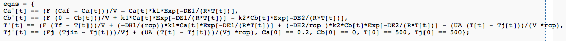6.) "Check the colors!"

Here is a list of font color that Mathmatica will output for a specific reason.

Local Variables in a certain Font Color

Local variables of Module and With in Green

1. Example:Function arguments and pattern names in Green (Italics)

1. Example:Variables made special by use in arguments in Turquoise

• Example:Errors and Warnings in a certain Font Color

Syntax Errors in Purple

• Example: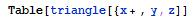Emphasized Syntax Errors in Dark Red with Yellow Background

• Example:Missing arguments in Bright Red

1. Example:Excess arguments in Bright Red

1. Example: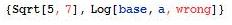Possible unwanted assignments in Bright Red

• Example: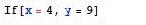Unrecognized option names in Bright Red

• Example: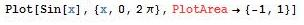Local Scope conflicts in Dark Red with Yellow Background

• Example:Variables that will go out of scope before being used in Bright Red

• Example:Shadowing in multiple contexts in Bright Red

• Example:Other in a certain Font Color

• Example:Strings in Dark Grey

• Example:Global symbols that have no value assigned in Bright Blue

• Example: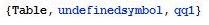## 7.10 Accessing Mathematica from your personal computer

If you prefer to use Mathematica from your home computer rather than one on campus, you can remotely log into a CAEN computer through U-M’s Virtual Sites service. Follow the steps outlined below:

2. The Virtual Sites page should appear on your screen. Click on the large, orange Connect Now button.
3. Under the heading What software do you need?, select Engineering (CAEN) Legacy XP. Then click on the Request Connection button toward the bottom of the page.
4. Follow the instructions under the heading Launch Virtual Sites by downloading and opening the file in the Internet Explorer information bar. A window will pop up on your screen titled Remote Desktop Connection. Click the Connect button.
5. You will then see the screen that appears when logging into a CAEN computer. Your unique name should already be included. Type your Kerberos password and click OK to log in to the CAEN computer.
6. Click OK on the Notice window that pops up and your personal settings will be applied. Also click OK if a System Requirements Wizard window appears.
7. If you scroll down using the scroll bar on the right-hand side of the screen, you will see the Start button. Click Start -> All Programs -> Math and Numerical Methods -> Wolfram Mathematica -> Mathematica 6. Once Mathematica opens on the computer screen, you may use it as though you were actually sitting in front of a CAEN computer on campus.
8. To log out, click Start -> Log Off. Under the Log Off Windows window, click Log Off.

## 7.11 References

• "Adams Method", Wolfram MathWorld, Online: August 5, 2007. Available http://mathworld.wolfram.com/AdamsMethod.html 
• "Gear Predictor-Corrector Method", Davidson University, Online August 5, 2007. Available http://webphysics.davidson.edu/Projects/SuFischer/node47.html 
• Fogler, H. Scott (2006), Elements of Chemical Reaction Engineering, New Jersey: Pretince Hall PTR. ISBN 0-13-047394-4